# RSI Filter Indicator for MT4

Introduction to the RSI Filter Indicator

The Relative Strength Index (RSI) is one of the most popular oscillator indicators. This is because it is a very versatile oscillator which is also very responsive to price action.

The RSI Filter Indicator was developed to improve on the standard RSI as it provides an objective indication of the trend direction with the addition of a signal line.

## What is the RSI Filter Indicator?

The RSI Filter is a momentum indicator which is based on the Relative Strength Index (RSI) with a slight modification which adds a signal line to the RSI.

This indicator presents the trend direction as an oscillator plotting two lines. The first line it plots is the main RSI line. This is the blue line with jagged oscillations. This line oscillates within the range of zero to 100. The second line, which is the smooth red line, is a signal line derived from the RSI line.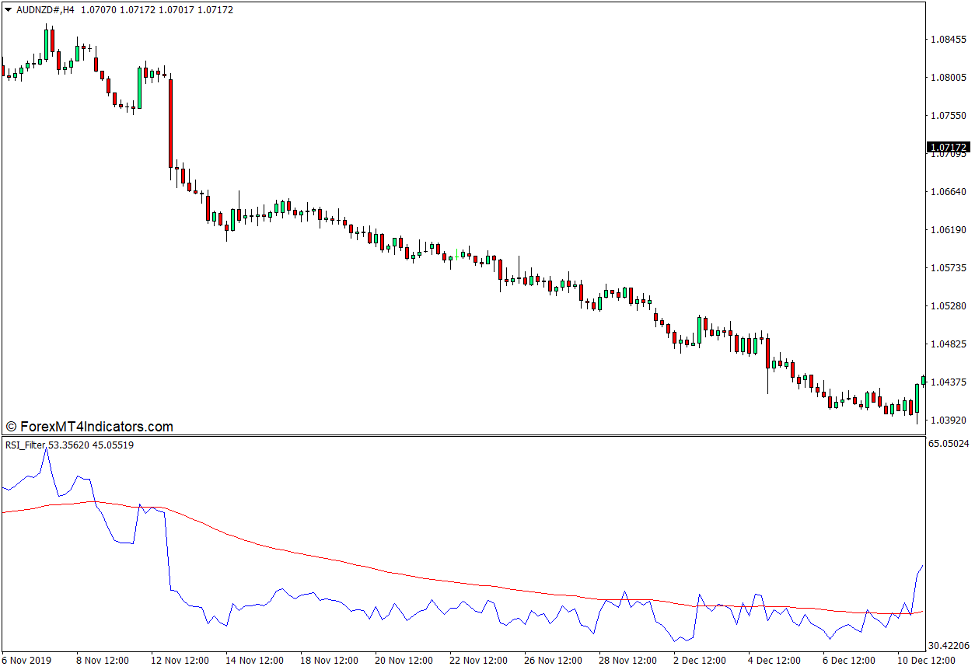Trend direction can be observed based on where the RSI line generally is in relation to the signal line. The RSI line is generally above the signal line during uptrends and below the signal line during downtrends.

## How the RSI Filter Indicator Works?

The RSI Filter Indicator uses the same calculations as the RSI oscillator with an added signal line.

This indicator uses the formula shown below.

RSI Step 1 = 100 – [100 / (1 + (Average Gain / Average Loss))]

RSI Step 2 = 100 – [100 / (1 + ((Previous Average Gain x 13) + Current Gain) / – ((Previous Average Loss x 13) + Current Loss))]

The resulting value is then plotted as a point on the RSI line oscillations.

The signal line is calculated as a moving average derived from the RSI line.

## How to use the RSI Filter Indicator for MT4

The RSI Filter Indicator has a few modifiable options within its indicator settings.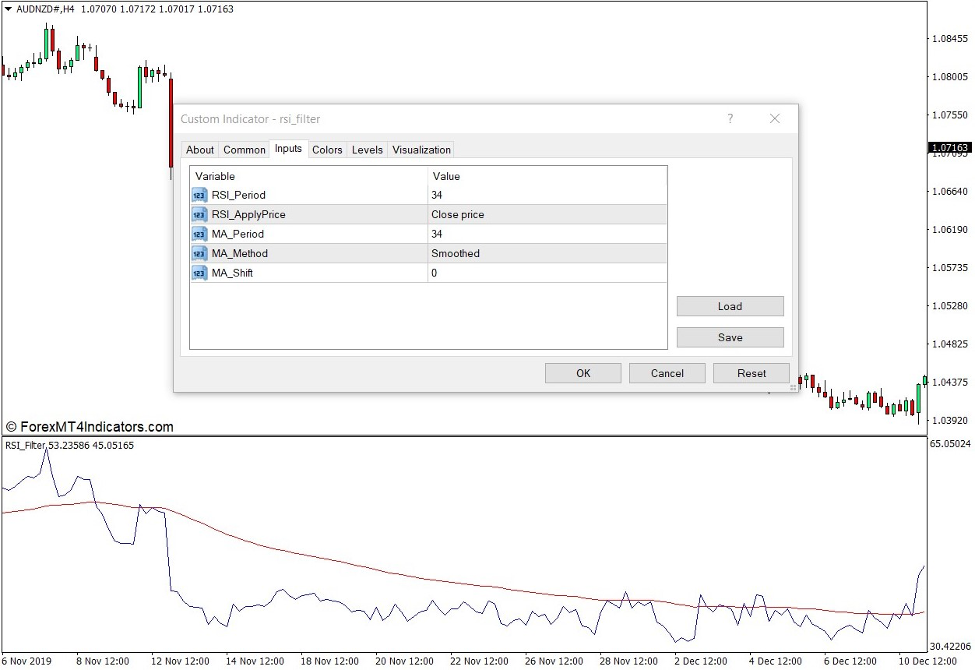“RSI_Period” allows users to modify the period length used to calculate for the RSI line.

“RSI_AppliedPrice” allows users to select the price point used to calculate for the RSI line.

“MA_Period” allows users to modify the period length used to calculate for the signal line.

“MA_Method” allows users to select the moving average method used to calculate for the signal line.

“MA_Shift” allows users to shift the signal line forward or back.

When to Enter?

Open a buy order if the RSI line crosses above its signal line in confluence with a bullish momentum candle.

When to Exit?

Close the trade as soon as the RSI line crosses below the signal line.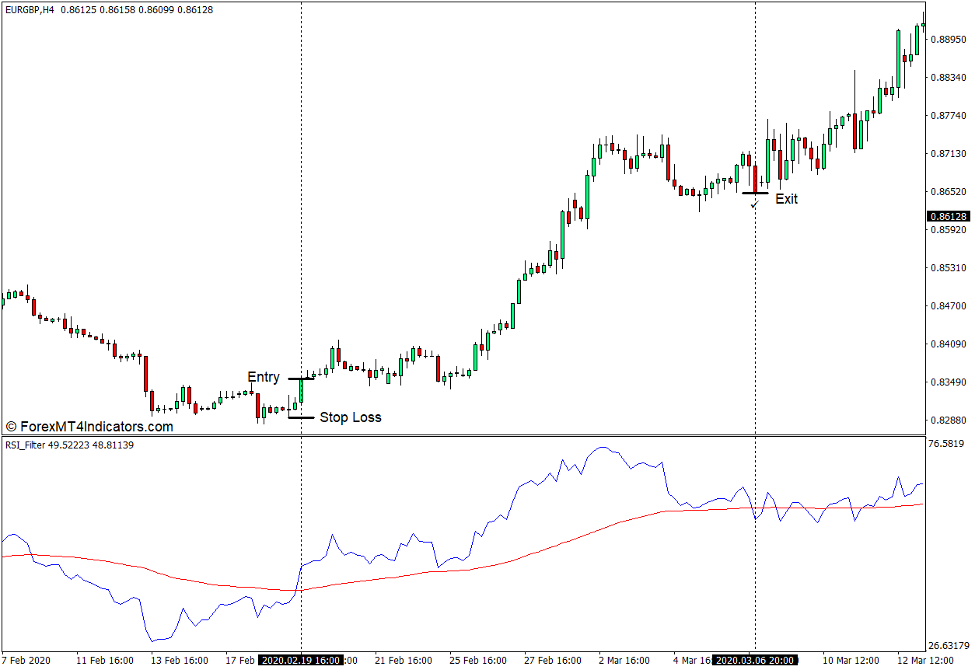When to Enter?

Open a sell order if the RSI line crosses below its signal line in confluence with a bearish momentum candle.

When to Exit?

Close the trade as soon as the RSI line crosses above the signal line.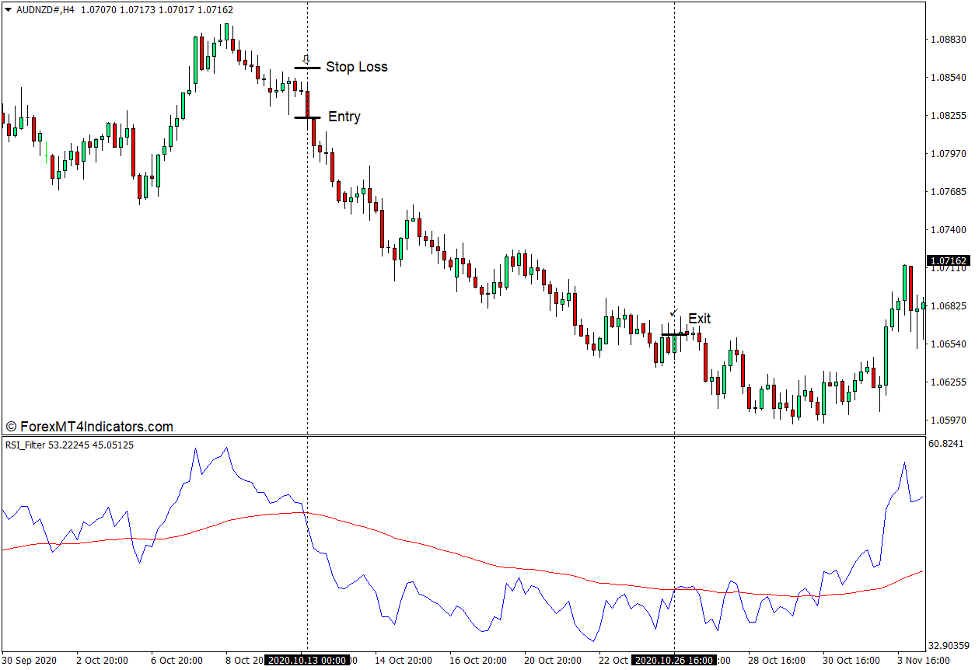## Conclusion

The RSI Filter Indicator can be used as a trend or momentum reversal signal indicator. However, it does produce a lot of false signals whenever the market is starting to create a tight and choppy market range. As such, it is best used only in confluence with other technical indications. It is also very effective as a trend direction filter wherein traders would trade exclusively in the direction where the RSI line is in relation to the signal line.

RSI Filter Indicator for MT4 is a Metatrader 4 (MT4) indicator and the essence of this technical indicator is to transform the accumulated history data.

RSI Filter Indicator for MT4 provides for an opportunity to detect various peculiarities and patterns in price dynamics which are invisible to the naked eye.

• Free \$50 To Start Trading Instantly! (Withdrawable Profit)
• Deposit Bonus up to \$5,000
• Unlimited Loyalty Program
• Award Winning Forex Broker
• Additional Exclusive Bonuses Throughout The Year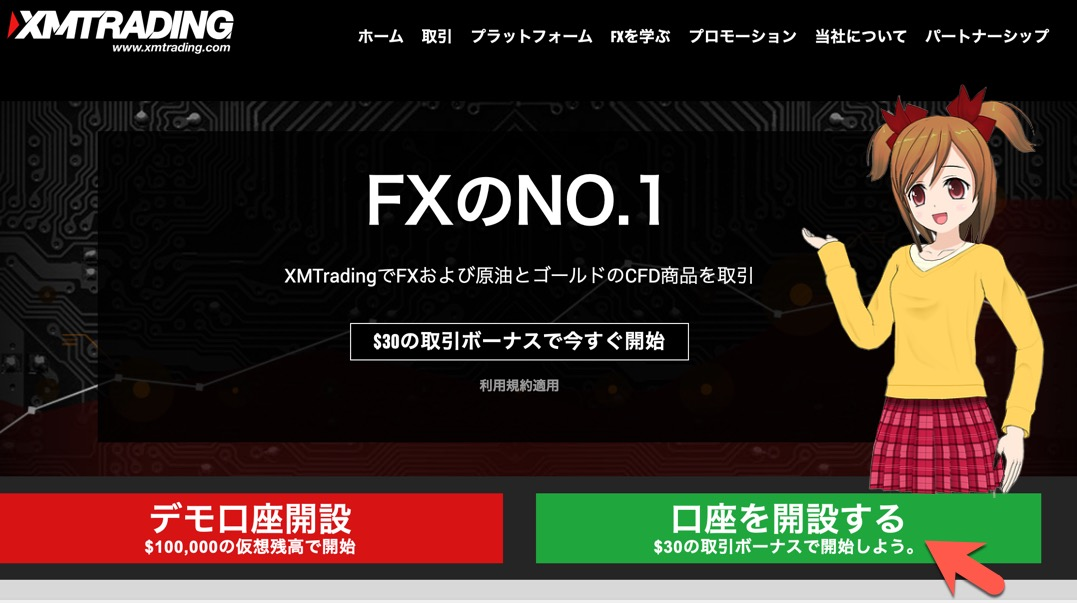#### How to install RSI Filter Indicator for MT4.mq4?

• Copy RSI Filter Indicator for MT4.mq4 to your Metatrader Directory / experts / indicators /
• Select Chart and Timeframe where you want to test your MT4 indicators
• Right click on RSI Filter Indicator for MT4.mq4
• Attach to a chart
• Modify settings or press ok
• Indicator RSI Filter Indicator for MT4.mq4 is available on your Chart

#### How to remove RSI Filter Indicator for MT4.mq4 from your Metatrader Chart?

• Select the Chart where is the Indicator running in your Metatrader 4 Client
• Right click into the Chart
• “Indicators list”
• Select the Indicator and delete